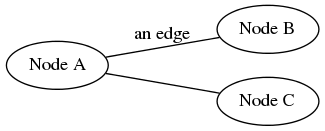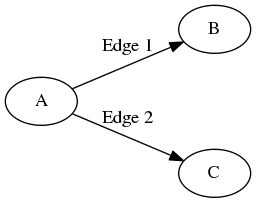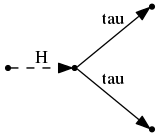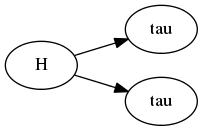# Some Theory: Graphs and Particle Representations¶

This is a brief introduction to graphs, some terminology, and how particle event trees can be represented by a graph.

## Basics¶

A graph is composed of a set of nodes, along with edges which connect those nodes. An example of a simple graph is shown below.Edges may have a direction associated with them; in this case we have a directed graph or digraph. An example of a digraph is shown below.A directed edge has an outgoing node (the one it leaves) and an incoming node (the one it is going into). In the example, Edge 1 has outgoing node A, and incoming node B, whilst Edge 2 has outgoing node A and incoming node C. This implies some sort of “relationship” between nodes.

## Graphs and the Representations of Particles¶

A particle event is characterised by the production and decay of particles; for example two gluons combine to make a Higgs boson, or a pion decays to two photons. There are parents (historically “mothers”) such as the gluons or pion, and children (“daughters”), the Higgs or photons, and the parents “produce” the children. Therefore, there are relationships between these particles, which we can denote using graphs in 2 distinct ways.

Physicists are already used to this concept without realising it: a Feynman diagram (see below) is a graph, where each particle is represented by an edge, and a node with incoming and outgoing particles indicates some interaction (a vertex).This association of particles to edges is deemed the edge representation. In this representation, nodes act as endpoints of edges, and a node with several incoming and outgoing particles may be interpreted as “all incoming particles are parents to all outgoing particles (children)”. That is, the incoming Higgs is parent to the two tau children.

This is not the only possible assignment of particles to a direct graph however. Whilst natural from a theoretical particle physics persepctive, one can pose an alternate formulation. If we choose to represent each particle as a node, then each parent-daughter relationships can be denoted with a directed edge. In the example above of Higgs decaying to a pair of taus, there are two (parent, child) relationships, (Higgs, tau), so we can denote this as:This is the node representation. Given a list of parent-child relationships, this is the natural representation, and is therefore the default for Pythia8, LHE, Heppy graphs.

## Conversion between representations¶

Naturally, one might ask if it is possible to convert between the two representations. Spoiler: yes! Although it is sometimes non-trivial.

### Edge to Node (easier)¶

Due to the definition of a node, this conversion is easy:

1. On our new graph, for each edge particle, create a node for that particle
2. For each vertex, iterate through all combinations of (incoming, outgoing) edges; for each draw a directed line between the corresponding pair of nodes.

And that’s it!

A simple example is shown:

### Node to Edge (harder)¶

Unlike edge to node, this is not always trivial. Consider the very simple example below.